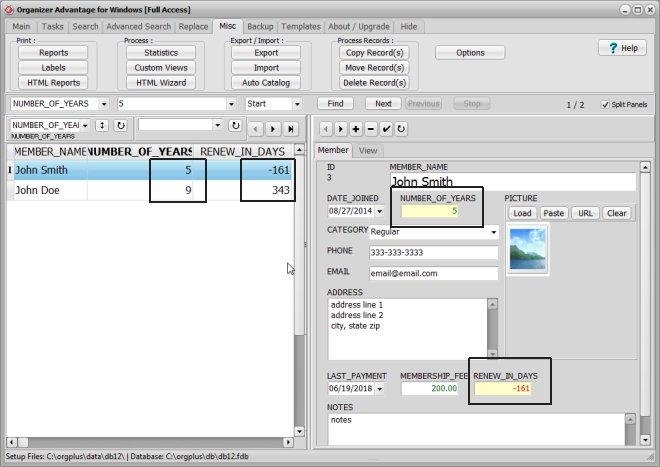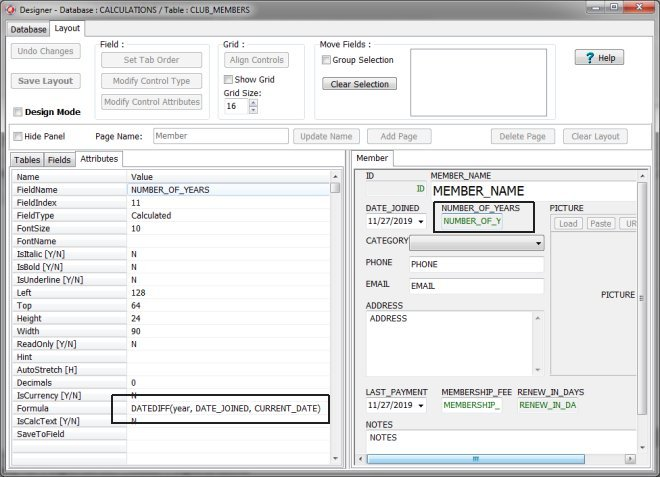﻿ Database Designer for Windows: designer data field calculated

### |  Organizer Advantage HowTo/Help  |  Designer HowTo/Help  |

#### Designer Help > Data Fields, Controls > Advanced > Calculated

easy-to-use database designer

## Calculated

Calculated data fields do not store the calculated value in the table. Calculated data fields are dynamic. The value of this field is recalculated for all records every time you load a table and only for the newly selected record. The value is calculated according to the arithmetic, string processing, or simple SQL query formula.

The program displays the value of this field on the record form, on the grid, in reports, statistics, or you can use this field in search or sort functions.

Use this field type in complex database solutions when modification of some values in the record and the use of variable parameters (current time, current year, current date, ...) requires dynamic recalculations.

Organizer Advantage is using Firebird SQL. In calculated data fields use Firebird operators or functions:

• arithmetic operators: +, -, *, /
• concatenation operator: || (double-pipe)
• constants: a number or a string enclosed in apostrophes
• functions: CURRENT_DATE, CURRENT_TIME, ...

If you want to use a calculated field in your table:

• Create Calculated field type
• Define calculation options: select data fields, define formula
• Note: MasterDetail, Calculated, and QueryEdit fields are virtual (dynamic) data fields, those fields are placed at the end of the table structure by the Designer, regardless of your field definition order.

In the below database sample there is a table: CLUB_MEMBERS.

• There are two calculated data fields: NUMBER_OF_YEARS, RENEW_IN_DAYS.
• Those fields are recalculated for every member record when you open CLUB_MEMBERS table.Copyright © 2021 · All Rights Reserved · PrimaSoft PC